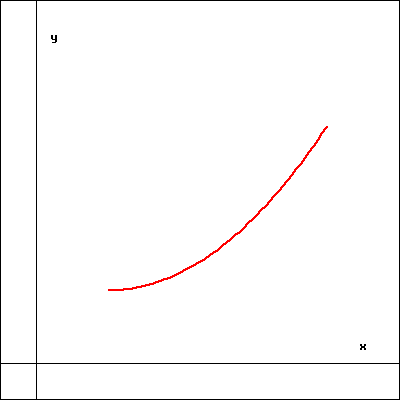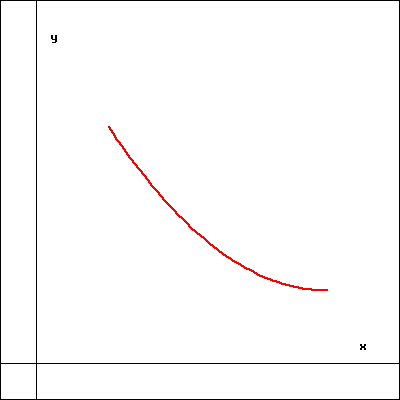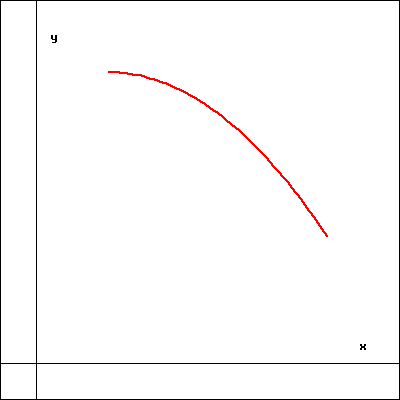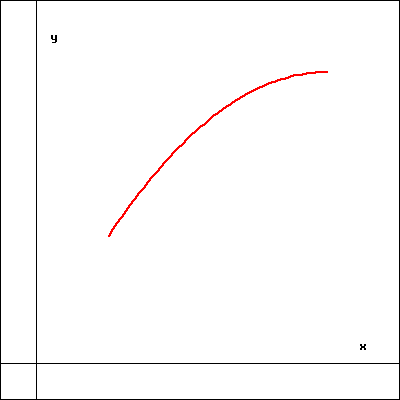Consider the function $f(x) = 2x^{6}-3x^{4}$.

The x-intercepts occur at $x=$
NOTE: If there are more than one, separate your answers by a comma.

There a y-intercept at $y =$

To analyze the shape of the graph of $f$ it helps to separate the domain into disjoint open intervals whose endpoints are critical points of $f$ or $f'$. Inside each interval there are no critical points of $f$ or $f'$, so $f'$ and $f''$ cannot change sign inside the interval.

$A =$
$B =$
$C =$
$D =$
$E =$

For each of the intervals listed below, select the curve best representing the shape of the graph of $f$ on that interval.

On ($-\infty,A$):
On ($A,B$):
On ($B,C$):
On ($C,D$):
On ($D,E$):
On ($E,\infty$):1 23 4

(Click on a graph to enlarge it.)

You can earn partial credit on this problem.